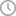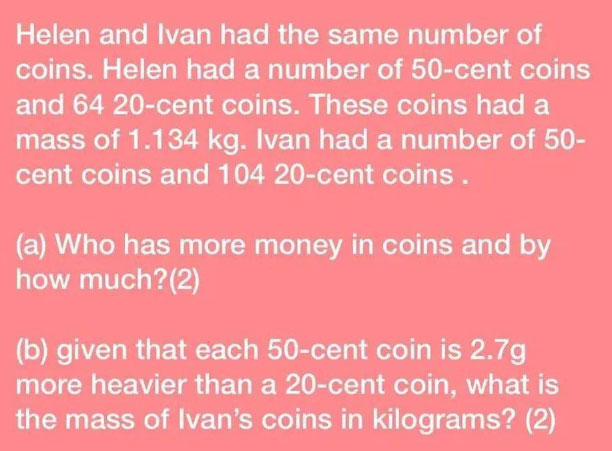# How to solve 2021 PSLE maths exam question about Helen's & Ivan's coins?

If adults were to take the PSLE again, a lot wouldn't make it.October 02, 2021, 06:00 AM

Sitting for the Primary School Leaving Examination is a scary experience, as anyone who has gone through it can attest.

In 2021, 12-year-old PSLE candidates across Singapore have the added pressure of taking their exams in the midst of Covid-19, as well as deal with a tough PSLE maths paper that has yet again left students in tears after it was administered on Friday, Oct. 1, according to The Straits Times.

This isn't the first time a PSLE maths paper has left students flustered and parents anxious and annoyed.

## Sample 4 marks PSLE maths question

One particular question, about coins, allegedly from this year's exam, has been making the rounds on the internet shortly after the maths paper ended.

How the question in its entirety came to be circulated online so quickly is not known, but needless to say, it has left otherwise intelligent adults flummoxed.Helen and Ivan had the same number of coins. Helen had a number of 50-cent coins, and 64 20-cent coins. These coins had a mass of 1.134kg. Ivan had a number of 50-cent coins and 104 20-cent coins.

(a) Who has more money in coins and by how much? (2)

(b) given that each 50-cent coin is 2.7g more heavier than a 20-cent coin, what is the mass of Ivan's coins in kilograms? (2)

Whether this was exactly how the question was phrased is not known, as it could have been regurgitated from memory and rewritten by an adult, which explains the grammatical errors.

But beyond the poor standard of English and the awkward sentence structure, the question is 100 per cent solvable -- and supposedly meant to be solved in a matter of minutes as it is only allocated 4 marks in total.

## How to solve it

If you are an adult who has been in the real world for a long time since leaving school, this question is akin to asking you to do a lot with very little information.

And the natural tendency is to try to find out how many coins in total both Helen and Ivan have -- which is the wrong way to go about it.

After sifting through several solutions on Facebook presented by many adults who attempted to solve it and show how they did it, here is one with graphs and clear logical thinking that nailed it.

As seen in the working below that doesn't use algebra, the key is to remember that Helen and Ivan have the same number of coins without knowing the total number of coins.

Since we know the number of 20-cent coins Helen and Ivan each have (64 vs 104), we can assume any number of 50-cent coins beyond 104 coins would cancel each other out because we want to find out who has more money and by how much, which is asking for the difference without knowing the total number of coins.

In other words, Helen's first 64 20-cent coins and Ivan's first 64 20-cent coins cancel each other out.

Both of their 50-cent coins beyond the 104 coins also cancel each other out.

This leaves Helen with 40 50-cent coins = \$20

And this leaves Ivan with 40 20-cent coins = \$8

The difference is, therefore, \$12.

And this second part is where adults stumble, especially if they are still preoccupied with wanting to know how many coins exactly does Helen and Ivan have.

Which is actually not necessary -- as long as you remember that the number of coins both Helen and Ivan have are the same and finite.

This math question is a logic question.

The trick is to see using the graph that Helen's coins are heavier overall than Ivan's coins as she has more 50-cent coins and fewer 20-cent coins as compared to Ivan.

This question can be solved without knowing the total number of coins and the weight of each coin.

(i) Since Helen's coins weigh 1.134kg or 1134g in total,

(ii) both Helen and Ivan have the same number of coins,

(iii) it follows that the difference in weight between Helen's and Ivan's coins is the weight difference between Helen's 40 50-cent coins and Ivan's 40 20-cent coins -- because the weight of the other coins cancel each other out.

To reiterate, since each 20-cent coin is 2.7g lighter, the student should be able to surmise that 40 20-cent coins weigh 108g less than 40 50-cent coins.

Hence, 1134 - 108 = 1026g or 1.026kg

## Adulting vs Taking PSLE again

If you utterly failed to grasp what is going on, it is suffice to say that the PSLE these days is indeed still very difficult, which explains the widespread gnashing of teeth post-exam, and a lot of adults wouldn't make it if they had to retake it again now.

Once again, back in the real world, the numbers 1134 and 1026 will probably be sold out for the 4D lottery draws this coming Saturday and Sunday.

Follow and listen to our podcast here